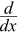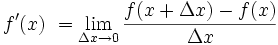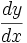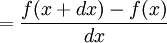# 导数入门

 坡度 = Y 的改变X 的改变我们可以求两点之间的 平均 坡度.但我们怎样求在一点的坡度？ 没有什么可以测量的！但是，在导数里，我们可以用一个很小的差…… ……然后把它缩小到零。## 求个导数！x 从 x 变到 x+Δx y 从 f(x) 变到 f(x+Δx)

• 代入这个坡度公式： ΔyΔx = f(x+Δx) − f(x)Δx
• 尽量简化
• Δx 缩小到零。

### 例子：函数 f(x) = x2

 开始： f(x+Δx) = (x+Δx)2 展开 (x + Δx)2: f(x+Δx) = x2 + 2x Δx + (Δx)2

 坡度公式是： f(x+Δx) − f(x) Δx 代入 f(x+Δx) 和 f(x)： x2 + 2x Δx + (Δx)2 − x2 Δx 简化 (x2 and −x2 约去）： 2x Δx + (Δx)2 Δx 再简化（除以 Δx）： = 2x + Δx 当 Δx 趋近 0时，我们得到： = 2xx2 = 2x
"x2 的导数等于 2x"
"x2 的 d dx 等于 2x"f’(x) = 2x
"f(x) 的导数等于 2x"

### 例子：x3是什么？

 开始： f(x+Δx) = (x+Δx)3 展开 (x + Δx)3: f(x+Δx) = x3 + 3x2 Δx + 3x (Δx)2 + (Δx)3

 坡度公式： f(x+Δx) − f(x) Δx 代入 f(x+Δx) 和d f(x)： x3 + 3x2 Δx + 3x (Δx)2 + (Δx)3 − x3 Δx 简化 （x3 and −x3 约去）： 3x2 Δx + 3x (Δx)2 + (Δx)3 Δx 再简化 （除以 Δx）： = 3x2 + 3x Δx + (Δx)2 当 Δx 趋近 0 时，我们得到：x3 = 3x2

## 记法

"缩小到零" 可以写一个 极限，像这样："f 的导数等于 当 Δx 趋近零时，f(x+Δx) - f(x) 除以 Δx的极限